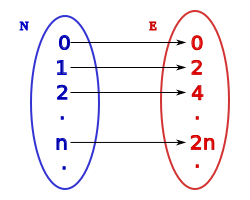# Almost Half Full!Let $S$ be the set of all natural numbers $n$ such that there are exactly $\lfloor \frac{n}{2}\rfloor$ prime numbers less than or equal to $n$.

What is the cardinality of the set $S$?

×